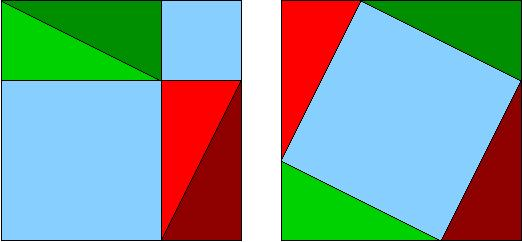## Why Does the Pythagorean Theorem work?

All of us had, in one time or another, encountered the Pythagorean Theorem. A fundamental formula in Geometry, the theorem was first found stated in Babylonian text around 1900 BC. It was around 500 BC, however, that a proof to the theorem was discovered. Traditionally, Phythagoras, a famous Greek mathematician, was believed to have found the proof. This claim, albeit credible, wasn’t thoroughly discussed in detail until the publication of Euclid’s Elements.

Euclid’s Proof

In Elements, Euclid gave two proofs for the theorem to work. One of these proofs describes the following diagram:

Let A, B, C be the vertices of a right triangle, with a right angle at A. Drop a perpendicular from A to the side opposite the hypotenuse in the square on the hypotenuse. That line divides the square on the hypotenuse into two rectangles, each having the same area as one of the two squares on the legs.

Based on this geometrical arrangement, the following assumptions are taken:

1. If two triangles have two sides of the one equal to two sides of the other, each to each, and the angles included by those sides equal, then the triangles are congruent. (Side – Angle – Side Theorem)
2. The area of a triangle is half the area of any parallelogram on the same base and having the same altitude.
3. The area of any square is equal to the product of two of its sides.
4. The area of any rectangle is equal to the product of two adjacent sides (follows from # 3).

The idea behind this proof is that the top squares are morphed into parallelograms with the same size, then turned and morphed into the left and right rectangles in the lower square, again at constant area.Algebraic and Geometric Proofs, But Not Trigonometric Proofs

In a book, The Pythagorean Proposition, by an early 20th century professor Elisha Scott Loomis, a collection of 367 proofs of the Pythagorean Theorem were proposed by the author. In the Foreward, the author rightly asserts that the number of algebraic proofs is limitless as is also the number of geometric proofs, but that the proposition admits no trigonometric proof.

Other Proofs By Other Authors

It is not just the Greek and other European scholars who have given proofs for the theorem. In India and China, the theorem has been proven using unique formulas. The Indian method is credited to the 12th century Hindu mathematician Bhaskara (Bhaskara II), while the Chinese method is found in the Chinese classic Chou Pei Suan Ching dated somewhere between 300 BC and 200 AD.

Why does it work?

No matter how many proofs were created, the formula will continue to prove that the theorem works. The reason for this is that the theorem is only applied to right triangles, and that all squares whose sides were used as basis were all perfect squares.

The theorem is reversible which means that a triangle whose sides satisfy a² + b² = c² is necessarily right angled. Euclid was the first to mention and prove this fact.

Categories: Mathematics